Next: 1.2.7 An Example: Up: 1.2 Complexity of Algorithms Previous: 1.2.5 Worst Case, Average Case, and Amortized Complexity

## 1.2.6 Big Omega and Big Theta Notations

The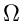notation specifies asymptotic lower bounds.

DEF. Big Omega. f (n) is said to be(g(n)) if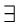a positive real constant C and a positive integer n0 such that

f (n)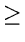Cg(n)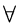nn0

An Alternative Definition : f (n) is said to be(g(n)) iffa positive real constant C such that

f (n)Cg(n)  for infinitely many values of n.

The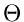notation describes asymptotic tight bounds.

DEF. Big Theta. f (n) is(g(n)) iffpositive real constants C1 and C2 and a positive integer n0, such that

C1g(n)f (n)C2g(n)nn0

eEL,CSA_Dept,IISc,Bangalore